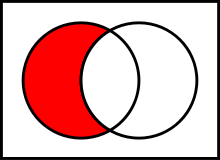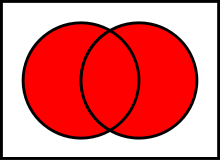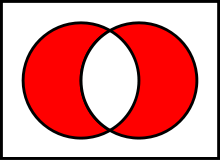# Binary operations with unordered sets

In this post I will do algorithmization of the array operations and describe the operations.

Summary:

• I. Intersection of sets
• II. Différence of sets
• III. Union of sets
• IV. Symmetric difference of sets
• conclusion

## I. Intersection of sets

The intersection of two sets A and B is such a sets that contains all elements of A that also belong to B (or equivalently, all elements of B that also belong to A), but no other elements.The time complexity of its calculation is `O(m*n)`, where `m` and `n` are the lengths of A and B sets.

``````function intersection(A, B)
{
var m = A.length, n = B.length, c = 0, C = [];
for (var i = 0; i < m; i++)
{
var j = 0, k = 0;
while (B[j] !== A[ i ] && j < n) j++;
while (C[k] !== A[ i ] && k < c) k++;
if (j != n && k == c) C[c++] = A[ i ];
}
return C;
}``````

For example,

``intersection ([1,2,3,7,9],[4,5,7,2,1,5]); // Output: [1,2,7]``

## II. Complement of sets

A complement of B in A is a set which contains all the elements of A which does not belong to B.The time complexity is `O(m*n)`, where `m` and `n` are the lengths of the operated sets.

``````function diff(A, B)
{
var M = A.length, N = B.length, c = 0, C = [];
for (var i = 0; i < M; i++)
{
var j = 0, k = 0;
while (B[j] !== A[ i ] && j < N) j++;
while (C[k] !== A[ i ] && k < c) k++;
if (j == N && k == c) C[c++] = A[ i ];
}
return C;
}``````

For example,

``````diff([1,2,3,7,9],[4,5,7,2,1,5]); // Output [3,9]
diff([4,5,7,2,1,5], [1,2,3,7,9]); // Output [4,5]``````

## III. Union of sets

The union of two sets A and B is the set of elements which are in A, in B, or in both A and B.The time complexity is `O(m*n)`, where `m` and `n` are the lengths of A and B sets.

``````function sum(A, B)
{
var M = A.length, N = B.length, count = 0, C = [];
C = A;
count = M;
var cnt = 0;
for (var i=0;i``````

`For example,`

``````sum([1,2,3,7,9],[4,5,7,2,1,5]); // Output [1,2,3,7,9,4,5]
sum([4,5,7,2,1,5],[1,2,3,7,9]); // Output [4,5,7,2,1,5,3,9]``````

## `IV. Symmetric difference of sets`

```The symmetric difference of two sets is the set of elements which are in either of the sets and not in their intersection.```

``

`The time complexity is O(2m*n), where m and n are the lengths of operated sets.`

`In fact, this is complement of arrays, first A \ B and then B \ A.`

``````function symmetricDiff(A,B)
{
var M = A.length, N = B.length, c = 0, C = [];
for (var i = 0; i < M; i++)
{
var j = 0, k = 0;
while (B[j] !== A[ i ] && j < N) j++;
while (C[k] !== A[ i ] && k < c) k++;
if (j == N && k == c) C[c++] = A[ i ];
}
for (var i = 0; i < N; i++)
{
var j = 0, k = 0;
while (A[j] !== B[ i ] && j < M) j++;
while (C[k] !== B[ i ] && k < c) k++;
if (j == M && k == c) C[c++] = B[ i ];
}
return C;
}``````

`For example,`

``````symmetricDiff([1,2,3,7,9],[4,5,7,2,1,5]);// Output: [3,9,4,5]
symmetricDiff([1,2,3,4,5],[3,4,5,6,7]); // Output: [1,2,6,7]``````

## `Conclusion`

`In practise this can be used, for example, to calculate which followers 2 users share in a social network.`

``` 7th October 2015 ```
``` [RU] Бинарные операции над неупорядоченными множествами Author: Roman Mestsheryakov GitHub: romkagolovadvayha Site: https://connected.su Translator: Varya Stepanova GitHub: varya Twitter: @varya_en Site: http://varya.me/ ```
``` Facebook Twitter Google+ If you've spotted a mistake, feel free to edit it on GitHub. (function(){ window.disqus_shortname = 'frontendbabel'; window.disqus_developer = '1'; window.disqus_url = 'http://frontendbabel.info/articles/binary-operations-with-irregular-sets'; window.disqus_identifier = 'articles-binary-operations-with-irregular-sets-index'; window.disqus_title = 'Binary operations with unordered sets'; if ( window.DISQUS ) { return DISQUS.reset({ reload: true, config: function () { this.page.identifier = window.disqus_identifier; this.page.url = window.disqus_url; this.page.title = window.disqus_title; } }); } else { var dsq = document.createElement('script'); dsq.type = 'text/javascript'; dsq.async = true; dsq.src = 'http://' + disqus_shortname + '.disqus.com/embed.js'; (document.getElementsByTagName('head') || document.getElementsByTagName('body')).appendChild(dsq); } })(); Please enable JavaScript to view the comments powered by Disqus. comments powered by Disqus ```
``` (function(i,s,o,g,r,a,m){i['GoogleAnalyticsObject']=r;i[r]=i[r]||function(){ (i[r].q=i[r].q||[]).push(arguments)},i[r].l=1*new Date();a=s.createElement(o), m=s.getElementsByTagName(o);a.async=1;a.src=g;m.parentNode.insertBefore(a,m) })(window,document,'script','//www.google-analytics.com/analytics.js','ga'); ga('create', 'UA-52228839-1', 'frontendbabel.info'); ga('send', 'pageview'); ```